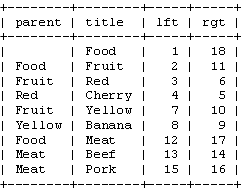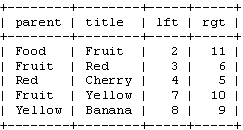# Storing Hierarchical Data in a Database, Part 2

Programming

Share:

#### Free JavaScript Book!Write powerful, clean and maintainable JavaScript.

RRP \$11.95

## Modified Preorder Tree Traversal

Now, let’s have a look at another method for storing trees. Recursion can be slow, so we would rather not use a recursive function. We’d also like to minimize the number of database queries. Preferably, we’d have just one query for each activity.

We’ll start by laying out our tree in a horizontal way. Start at the root node (‘Food’), and write a 1 to its left. Follow the tree to ‘Fruit’ and write a 2 next to it. In this way, you walk (traverse) along the edges of the tree while writing a number on the left and right side of each node. The last number is written at the right side of the ‘Food’ node. In this image, you can see the whole numbered tree, and a few arrows to indicate the numbering order.We’ll call these numbers left and right (e.g. the left value of ‘Food’ is 1, the right value is 18). As you can see, these numbers indicate the relationship between each node. Because ‘Red’ has the numbers 3 and 6, it is a descendant of the 1-18 ‘Food’ node. In the same way, we can say that all nodes with left values greater than 2 and right values less than 11, are descendants of 2-11 ‘Fruit’. The tree structure is now stored in the left and right values. This method of walking around the tree and counting nodes is called the ‘modified preorder tree traversal’ algorithm.

Before we continue, let’s see how these values look in our table:Note that the words ‘left’ and ‘right’ have a special meaning in SQL. Therefore, we’ll have to use ‘lft’ and ‘rgt’ to identify the columns. Also note that we don’t really need the ‘parent’ column anymore. We now have the lft and rgt values to store the tree structure.

Retrieve the Tree

If you want to display the tree using a table with left and right values, you’ll first have to identify the nodes that you want to retrieve. For example, if you want the ‘Fruit’ subtree, you’ll have to select only the nodes with a left value between 2 and 11. In SQL, that would be:

``SELECT * FROM tree WHERE lft BETWEEN 2 AND 11;``

That returns:Well, there it is: a whole tree in one query. To display this tree like we did our recursive function, we’ll have to add an ORDER BY clause to this query. If you add and delete rows from your table, your table probably won’t be in the right order. We should therefore order the rows by their left value.

``SELECT * FROM tree WHERE lft BETWEEN 2 AND 11 ORDER BY lft ASC;``

The only problem left is the indentation.

To show the tree structure, children should be indented slightly more than their parent. We can do this by keeping a stack of right values. Each time you start with the children of a node, you add the right value of that node to the stack. You know that all children of that node have a right value that is less than the right value of the parent, so by comparing the right value of the current node with the last right node in the stack, you can see if you’re still displaying the children of that parent. When you’re finished displaying a node, you remove its right value from the stack. If you count the elements in the stack, you’ll get the level of the current node.

``````<?php
function display_tree(\$root) {
// retrieve the left and right value of the \$root node
\$result = mysql_query('SELECT lft, rgt FROM tree '.
'WHERE title="'.\$root.'";');
\$row = mysql_fetch_array(\$result);

\$right = array();

// now, retrieve all descendants of the \$root node
\$result = mysql_query('SELECT title, lft, rgt FROM tree '.
'WHERE lft BETWEEN '.\$row['lft'].' AND '.
\$row['rgt'].' ORDER BY lft ASC;');

// display each row
while (\$row = mysql_fetch_array(\$result)) {
// only check stack if there is one
if (count(\$right)>0) {
// check if we should remove a node from the stack
while (\$right[count(\$right)-1]<\$row['rgt']) {
array_pop(\$right);
}
}

// display indented node title
echo str_repeat('  ',count(\$right)).\$row['title']."n";

// add this node to the stack
\$right[] = \$row['rgt'];
}
}
?>``````

If you run this code, you’ll get exactly the same tree as with the recursive function discussed above. Our new function will probably be faster: it isn’t recursive and it only uses two queries.

The Path to a Node

With this new algorithm, we’ll also have to find a new way to get the path to a specific node. To get this path, we’ll need a list of all ancestors of that node.

With our new table structure, that really isn’t much work. When you look at, for example, the 4-5 ‘Cherry’ node, you’ll see that the left values of all ancestors are less than 4, while all right values are greater than 5. To get all ancestors, we can use this query:

``SELECT title FROM tree WHERE lft < 4 AND rgt > 5 ORDER BY lft ASC;``

Note that, just like in our previous query, we have to use an ORDER BY clause to sort the nodes. This query will return:

``````+-------+
| title |
+-------+
| Food  |
| Fruit |
| Red   |
+-------+``````

We now only have to join the rows to get the path to ‘Cherry’.

How Many Descendants

If you give me the left and right values of a node, I can tell you how many descendants it has by using a little math.

As each descendant increments the right value of the node with 2, the number of descendants can be calculated with:

``descendants = (right â€“ left - 1) / 2``

With this simple formula, I can tell you that the 2-11 ‘Fruit’ node has 4 descendant nodes and that the 8-9 ‘Banana’ node is just a child, not a parent.

Go to page: 1 | 2 | 3### Gijs Van Tulder

Gijs is a full time Dutch student in economics and a spare time Web developer. He spends his time developing scripts using PHP, MySQL and other external programs. Visit him at http://gvtulder.f2o.org/

#### New books out now!